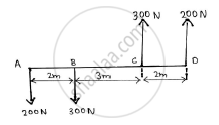# Find the Resultant of the Force System as Shown in the Given Figure. - Engineering Mechanics

Find the resultant of the force system as shown in the given figure.#### Solution

Taking forces having direction upwards as positive. Net force = 200+300-200-300

=0 N
Taking moments of the forces about the point A Taking anticlockwise moment direction as positive
∴ MA = 200 x7 +300 x 5 – 300 x2
=2300 Nm (anticlockwise direction)

The resultant force is 0.
Net moment is 2300 Nm(anticlockwise)

Concept: Resultant of concurrent forces
Is there an error in this question or solution?